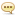Tutorials :

# Electric Circuits for Electrical Engineering and Electronics

Author: DownTR.CC   |   26 October 2020   |: 0MP4 | Video: h264, 1280x720 | Audio: AAC, 44.1 KHz, 2 Ch
Genre: eLearning | Language: English + .srt | Duration: 67 lectures (6h 1m) | Size: 1 GB

Start your career in electrical & electronics engineering by learning everything about electric circuits and electronics

What you'll learn:
Electrical Engineering fundamentals
Electronics fundamentals
What are electric circuits and electronics and the fundamental quantities in circuits like voltage and current
The differences between direct current (DC) and alternating current (AC)
What resistors, capacitors, and inductors are and how they are used in electric circuits and electronics
Sources of electricity in electric circuits and electronics
Simplification of resistive, capacitive, and inductive circuits
Basic electric circuit analysis techniques using Kirchhoff's voltage and current laws, nodal analysis, and loop analysis
Advanced electric circuit analysis techniques such as the superposition theorem, Thévenin's theorem, and Norton's theorem
Analysis of first-order electric circuits during transients
Concepts for AC electric circuit analysis such as admittance and reactance
Complete analysis of AC electric circuits
Electric circuit analysis techniques used in different electrical engineering and electronics engineering fields, such as analog electronics, digital electronics, and power electronics
Electric circuit analysis techniques needed for developing electronics with development boards such as the Arduino or Raspberry Pi

Requirements
Basic knowledge of algebra

Description
This course is designed to provide a complete overview of electric circuit analysis used in electrical engineering and electronics engineering. Electric circuit analysis is the most fundamental concept for electrical engineering, electronics engineering, and computer engineering. It is for that reason that electric circuit analysis is usually the first course taught in electrical, electronics, and computer engineering programs at universities, as basically anything related to electrical, electronics, or computer engineering stems from electric circuit analysis.

In this course you will learn everything about electric circuits and electronics, from the basics such as what an electric circuit is and the fundamentals of electrical quantities like voltage, current, and power, all the way to complex techniques for analyzing electric and electronics circuits. The course is roughly divided into the following sections:

1. Fundamentals of Electric Circuits and Electronics: in sections 2 and 3 of the course, we will discuss what an electric circuit is at the most basic level, followed by explanations of electrical quantities and sources of electricity. This is the foundation for electrical engineering and electronics engineering.

2. Basic DC Electric Circuit Analysis: in sections 4, 5, and 6 we will discuss the analysis of direct current (DC) circuits, starting from basic analysis techniques such as Kirchhoff's voltage law and Kirchhoff's current law (KVL and KCL), voltage division, current division, nodal analysis, and loop analysis. We will also be discussing how complex resistive circuits can be simplified into equivalent circuits for easier analysis of electric circuits and electronics.

3. Advanced DC Electric Circuit Analysis: in section 7, we will discuss advanced analysis techniques for electric circuits and electronics such as the superposition theorem, Thevenin's theorem, and Norton's theorem.

4. Energy-Storing Devices in Electric Circuits and Electronics: in sections 8, and 9, we will discuss passive components in circuits that are able to store energy: capacitors and inductors. We will cover the fundamentals of capacitors and inductors, how they store energy, and how to simplify complex circuits containing combinations of capacitors and inductors into simpler circuits for easier analysis of electric circuits and electronics.

5. Transients in Electric Circuits and Electronics: in section 10, we will discuss the analysis of first-order electric circuits during transients. This is where things start getting a bit more advanced, but we will solve several examples to illustrate how circuits behave during transients, as opposed to the stead-state circuits previously discussed.

6. AC Electric Circuit Analysis: in section 11, we will discuss the analysis of alternating current (AC) electric circuits, starting from explaining how resistors, capacitors, and inductors behave in AC circuits (as opposed to DC circuits previously discussed) and moving into more complex topics such as defining new concepts like admittance and reactance and analyzing power dissipation in circuits.

In each section, several examples are solved to illustrate how to analyze practical circuits.

By learning all the fundamentals about electric circuit analysis and electronics, you will be able to continue studying other topics in electrical engineering, electronics engineering, and computer engineering, such as analog electronics, digital electronics, circuit design, electric machines, power systems, and more.

Remember that Udemy offers a 30-day money-back guarantee. I am also always available for questions while you go through the course to ensure everything is clear.

See you in the course!

Who this course is for
Anybody with an interest in learning about electrical, electronics, or computer engineering. This is an introductory course, no prior engineering experience is required.
Homepage
https://www.udemy.com/course/electrical-engineering-dc-circuit-analysis/